# Compare Whole Numbers

##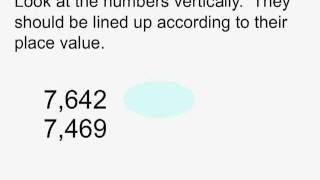By MathNook

MathNook presents Compare Whole Numbers an educational video resource on math.# Comparing and Ordering Whole Numbers

##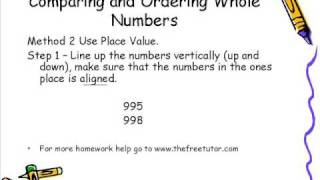By TheFreeTutor

This video describes and explains some easy steps for comparing and ordering whole numbers. This is a middle school math skill that should be mastered by the sixth grade.# Comparing three-digit numbers

##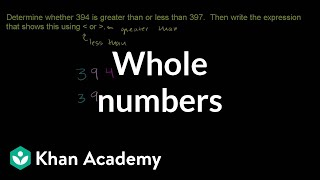By Khan Academy

Learn how to compare whole numbers. The numbers used in this video are 394 and 397.##By Lumos Learning

Subscribe Now: http://www.youtube.com/subscription_c...​ Watch More: http://www.youtube.com/ehoweducation​ Comparing whole numbers involves working with anything valued at zero and above. Learn about comparing whole numbers in grade five math with help from a professional private tutor in this free video clip. Expert: Rachel Kaplove Filmmaker: Alexis Guerreros Series Description: Most mathematical concepts really aren't that difficult, but you have the have the proper instruction. Get tips on math with help from a professional private tutor in this free video series.# Multiplying Fractions by Whole Numbers

##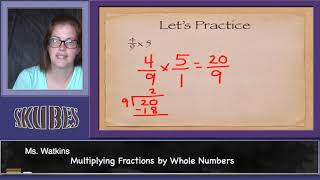By Skubes ed

Understand a multiple of a/b as a multiple of 1/b, and use this understanding to multiply a fraction by a whole number.# Multiplying Fractions by Whole Numbers 9-9

##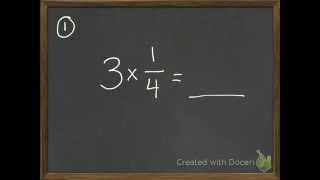By David Horan

multiply a fraction with a whole number# Multiplying Fractions by Whole Numbers 9-9

##By David Horan

multiply a fraction with a whole number.# Subtracting 6 Digit Whole Numbers without Regrouping

##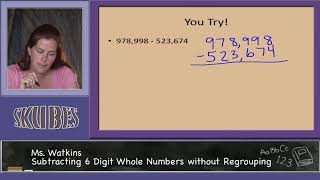By Skubes ed

Fluently add and subtract multi-digit whole numbers using the standard algorithm# Fractions to whole numbers (3.NF.3C)

##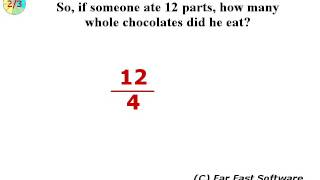By Far East Software

Express whole numbers as fractions, and recognize fractions that are equivalent to whole numbers.# Rounding Whole Numbers 3

##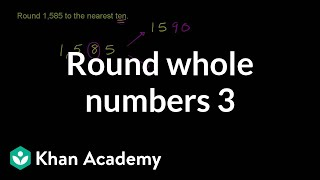By Khan Academy

You're becoming a pro at rounding whole numbers. Here's another example exercise. Can you do it first before watching the end of the video?# Approximating square roots

##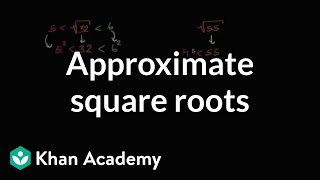By Khan Academy

Learn how to find the approximate values of square roots. The examples used in this video are √32, √55, and √123. The technique used is to compare the squares of whole numbers to the number we're taking the square root of.# Multiply fractions by whole numbers

##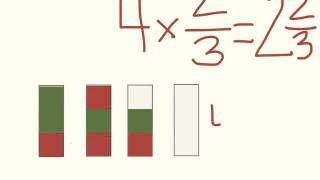By Gregory Asplund

Mutliplication of whole numbers# [3.NF.3c-1.0] Whole Numbers as Fractions - Common Core Standard

##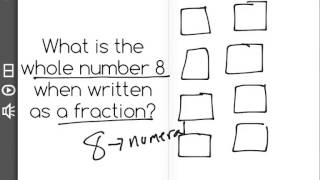By Freckle education

Explain equivalence of fractions in special cases. Express whole numbers as fractions, and recognize fractions that are equivalent to whole numbers.# eSpark Learning: Round Whole Numbers to the Nearest 10 or 100 (3. NBT, Quest 2)

##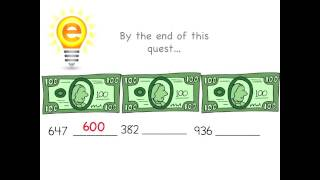By espark learning videos

use knowledge of place value to round whole numbers to the nearest 10 or 100.# eSpark Learning: Round Whole Numbers to the nearest 10 or 100 (3 NBT, Quest 1)

##By espark learning videos

Standard 3.NBT: Use place value understanding to round whole numbers to the nearest 10 or 100.# Dividing a whole number by a fraction

##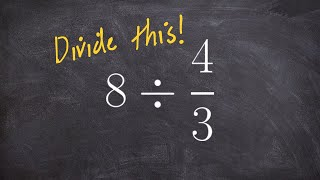By Brian Mclogan

To divide two fractions, we multiply the first fraction by the reciprocal of the second fraction.# Multiplying fractions by whole numbers 4.NF.B.4 4.NF.4

##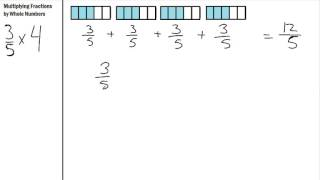By S Hart

Multiplying Fractions by Whole numbers using repeated addition# Multiplying Whole Numbers with Fractions

##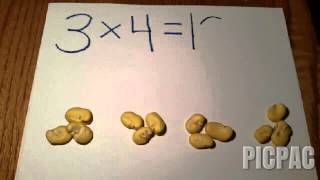By

Understand a fraction a/b as a multiple of 1/b# Grades 3rd to 5th ~ Multi-step Word Problems: Whole Numbers

##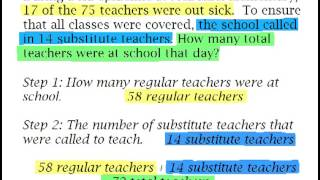By HYPE math

Word problems for grades 3rd - 5th that take more than one step to solve# Multiplying fractions by whole numbers word problem | Math | 4th grade | Khan Academy

##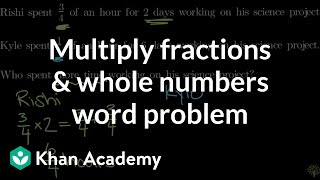By Khan Academy

solves a word problem that involves multiplying a fraction by a whole number